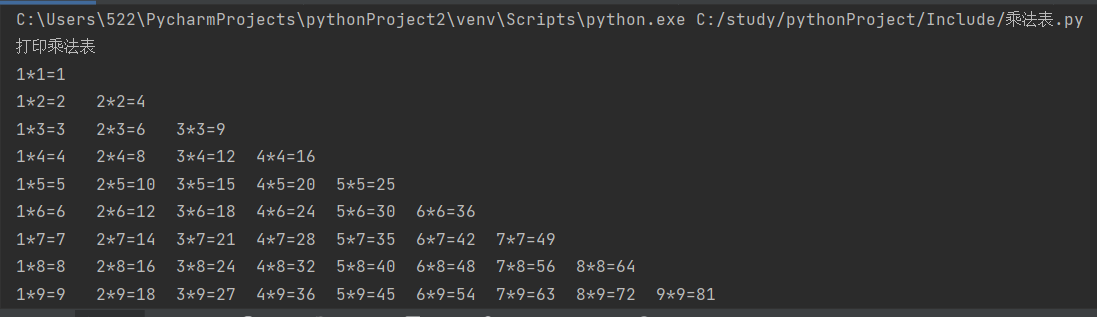# 一，双层for循环

``#    方法一print('打印乘法表')for i in range(1,10):   for j in range(1,i+1):   print('%d*%d=%d'%(j,i,i*j),end='\t') print('')``2，%d*%d=%d,是字符串的格式化（这里的格式化并不是全部清除，恢复出厂设置那样，而是规定了格式，让他输出的结果按照规定的格式）

3，end=”/t”是换行的意思，即每循环一次，换一次行。

# 二，双层while循环

``#方法二print('打印乘法表')i = 1while i <= 9:    j = 1    while (j <= i):        print(f'{i}*{j}={i * j}', end='\t')        j += 1    print('')    i += 1``1，j+=1表示每次训环j的值就增加一

2,f'{i}*{j}={i * j}’是字符串的格式化，f是转换为浮点数类型。

# 三，综合法

``#方法三print("打印九九乘法表：")for i in range(1, 10):    for j in range(1, i + 1):        print(f'{i}*{j}={i * j}', end='\t')    print()``

1，先是循环的是i，此时j为1，当i循环到9时，开启下一次循环，j的范围变为（1，3），但取不到3，并开始j的下一次循环为2，之后i在从1循环到9，在开启新的循环，以此类推。